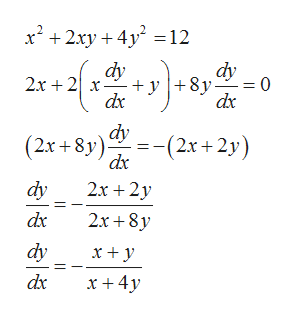Question
3 views

Use implicit differentiation to find an equation of the tangent line to the curve at the given point.

The equation is provided as the attached image.

check_circle

Step 1

Given equation of curve is

Step 2

We differentiate both sides of equation with respect to xhelp_outlineImage Transcriptionclosex2 2xy 12 dy 2x 2x +y8y dx dy dx dy (2x + 2y) dx (2x+8y) 2х + 2y dy 2x+8y dx dy x y x + 4y dx fullscreen
Step 3

Slope of tangent to curve f(x,y)=0 at point ...

### Want to see the full answer?

See Solution

#### Want to see this answer and more?

Solutions are written by subject experts who are available 24/7. Questions are typically answered within 1 hour.*

See Solution
*Response times may vary by subject and question.
Tagged in

### Derivative